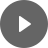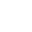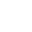10.1 Linear Equations - StudyNinja
Select Page

HSC Standard Maths Resources

Browse: 1. Home  »  10. Linear Relationship »  10.1 Linear Equations

10.1 Linear Equations

What is a linear equation? To begin with graphically, anything that produces straight line on a graph has got a linear equation behind it.

All of the following have linear equations behind them:x and y are the variables that are plotted on x and y axis, m is the gradient and c is the y-intercept. These will become clearer when we look at examples below.

Before looking into examples, note the following formula we will use to work out m and c:Example 1

James sells laptops. If he sells 10 laptops his profit is \$1,300 and when he sells 25 laptops his profit is \$3,550. You are told this follows linear equation. Draw this on a graph. What is the equation of his profit? Are there any limitations?

Example 2

What is the gradient and y-intercept of following linear equations?Example 31. What is the y-intercept of blue line?
2. Is gradient of red line positive or negative?
3. Between blue and green line, which one has higher gradient?
4. What is the gradient of yellow line?

Get access to 20 Mock Exams with over 700 exam-style questions for HSC Standard Maths.

You might find the following videos helpful related to this section:5A Linear Functions (1 of 5)

by Mr Bodgers (click to view channel)

Explains the difference between dependent and independent variables.5A Linear Functions (2 of 5)

by Mr Bodgers (click to view channel)

Explains how to draw a graph using a table of values.5A Linear Functions (3 of 5)

by Mr Bodgers (click to view channel)

Explains how to calculate the gradient and y-intercept by interpreting a graph.5A Linear Functions (4 of 5)

by Mr Bodgers (click to view channel)

Explains how to sketch a graph using the gradient-intercept method.5A Linear Functions (5 of 5)

by Mr Bodgers (click to view channel)

Explains how to recognise two equations that make parallel lines when graphed.5B Linear Models (1 of 2)

by Mr Bodgers (click to view channel)

How to graph a linear model involving electricity costs that include a service charge.5B Linear Models (2 of 2)

by Mr Bodgers (click to view channel)

How to graph a linear model involving depreciation of a vehicle.The following are the type of questions you can expect in exam:

Study notes of this section and other resources can be accessed here:

Browse: 1. Home  »  10. Linear Relationship »  10.1 Linear Equations